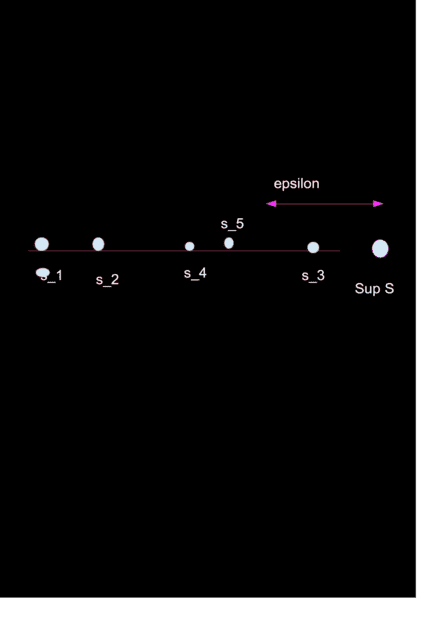# Proving that there is a sequence in S, such that ##\lim s_n = \sup S##

• Hall
In summary: For very ##n## there exists an ##s## such that $$\sup S - a_n \lt s \lt \sup S$$(Where ##a_n## is mono tonic decreasing sequence) implies that ##s \in S##, all these ##s## may form a sequence. But there can exist many points between ##\sup S -a_n ## and ##\sup S##, not just one ##s##.But there can exist many points between ##\sup S -a_n ## and ##\sup S##, not just one ##s##.

#### Hall

Homework Statement
Let S be a bounded nonempty subset of R such that sup S is not in S. Prove there is a sequence (sn) of points in S such that lim sn = sup S.
Relevant Equations
sup S is the least upper bound.
Let ##S=\{s_n:n∈N\}##. ##\sup S## is the least upper bound of S. For any ϵ>0, we have an m such that
##\sup S−\epsilon \lt s_m##
##\sup S−s_m \lt \varepsilon##
##|\sup S−s_m| \lt \varepsilon##

I mean to say that, no matter how small ϵ is, there is always an element of S whose distance from supS is less than that of ϵ, therefore there does exist a set of points which converge to supS, but I'm unable to get a thing like ##n \gt N \implies |\sup S−s_n| \lt \varepsilon##. Because, assume for a given ϵ, ##s_3## lies within ϵ of ##\sup S##, but ##s_4,s_5⋯s_n## all lies beyond the coverage of ##\epsilon##You only need to show the existence of one sequence. It would be nice if you could just set $s_n = \sup S - 2^{-n}$ but those points might not actually be in $S$. But you could use them as lower bounds for $s_n$...

Hall said:
Let ##S=\{s_n:n∈N\}##. ##\sup S## is the least upper bound of S. For any ϵ>0, we have an m such that
Are you trying to prove that every sequence in ##S## converges to ##\sup S##?

pasmith said:
You only need to show the existence of one sequence. It would be nice if you could just set $s_n = \sup S - 2^{-n}$ but those points might not actually be in $S$. But you could use them as lower bounds for $s_n$...
That’s a good hint. How about
$$s_n = \sup S - \frac{1}{n}$$?

as n grows, 1/n will get smaller and smaller and thus converging to ##\sup S##.

Hall said:
That’s a good hint. How about
$$s_n = \sup S - \frac{1}{n}$$?
How do you know that ##s_n \in S##?

Hall said:
That’s a good hint. How about
$$s_n = \sup S - \frac{1}{n}$$?

as n grows, 1/n will get smaller and smaller and thus converging to ##\sup S##.

You can take any strictly positive, strictly decreasing sequence $(a_n)$ whose limit is 0, but you can never be certain that $\sup S - a_n \in S$ for every $n \in \mathbb{N}$. However, since $\sup S - a_n < \sup S$ you do know that for every $n \in \mathbb{N}$ there exists an $s \in S$ such that $\sup S - a_n < s < \sup S$. What can you do with this observation?

pasmith said:
You can take any strictly positive, strictly decreasing sequence $(a_n)$ whose limit is 0, but you can never be certain that $\sup S - a_n \in S$ for every $n \in \mathbb{N}$. However, since $\sup S - a_n < \sup S$ you do know that for every $n \in \mathbb{N}$ there exists an $s \in S$ such that $\sup S - a_n < s < \sup S$. What can you do with this observation?
For very ##n## there exists an ##s## such that
$$\sup S - a_n \lt s \lt \sup S$$
(Where ##a_n## is mono tonic decreasing sequence) implies that ##s \in S##, all these ##s## may form a sequence.

But there can exist many points between ##\sup S -a_n ## and ##\sup S##, not just one ##s##.

Hall said:
But there can exist many points between ##\sup S -a_n ## and ##\sup S##, not just one ##s##.

Choose one of them.

•PeroK